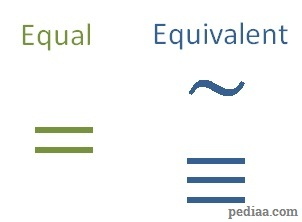# Difference Between Equal and Equivalent

## Main Difference – Equal vs. Equivalent

Equal and equivalent are terms that are used frequently in mathematics. The main difference between equal and equivalent is that the term equal refers to things that are similar in all aspects, whereas the term equivalent refers to things that are similar in a particular aspect. Note that in the set theory, the words “equal” and “equivalent” have specific meanings, as we will see below.

## What Does Equal Mean

In general, two things are equal if they are similar in all respects.

In the case of set theory, two sets are equal if they both contain the same elements. The order in which they are listed in a set does not matter. For instance, suppose$A=\left \{ 1,2,3,4 \right \}$ and$B=\left \{ 2,4,3,1 \right \}$ then,

the set$A$ is equal to the set$B$.

## What Does Equivalent Mean

Two things can be said to be equivalent if they are similar under a particular condition. Therefore, whether two entities are equivalent largely depends on the condition we use to  describe their equivalence. For instance, numbers 2 and 7 are equivalent in the sense that they are both prime numbers. However, if the condition we are interested in is finding out whether numbers are even, then in this sense 2 and 7 are not equivalent. We use the symbols$X\sim Y$ or$X\equiv Y$ to indicate that$X$ and$Y$ are equivalent.

Once a criterion has been defined, things that are equivalent satisfy the equivalence relations:

1. Reflexivity:$X\sim X$
2. Symmetry:  If$X\sim Y$, then$Y\sim X$
3. Transitivity: If$X\sim Y$ and$Y\sim Z$, then$X\sim Z$

In set theory, two sets are equivalent if they have the same number of elements. The elements themselves do not need to be the same, either, only the number of elements needs to be the same. For instance, suppose$C=\left \{ 1,2,3,4 \right \}$ and$D=\left \{ 8,2,7,150 \right \}$ then,

the sets$C$ and$D$ are equivalent.Symbols for expressing equality and equivalence

## Difference Between Equal and Equivalent

### General Meaning

When two things are equal, they are similar in all aspects.

When things are equivalent, they are similar in a particular aspect.

### In Set Theory

When two sets are equal, they contain the same elements.

When two sets are equivalent, they contain the same number of elements.